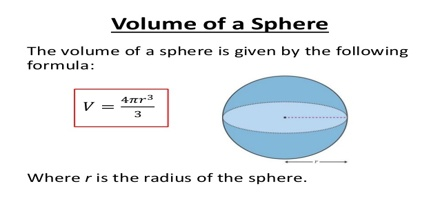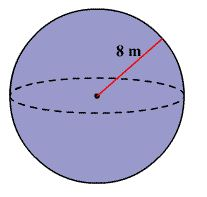Volume of a sphere means the number of cubic units that will exactly fill a sphere. The volume of a sphere can be found by the formula:

volume = 4/3 π r3

Where r is the radius of the sphere. The volume of a 3-dimensional solid is the amount of space it occupies.  Volume is measured in cubic units (in3, ft3, cm3, m3 etc cetera).  Be sure that all of the measurements are in the same unit before computing the volume.This formula was discovered over two thousand years ago by the Greek philosopher Archimedes. He also realized that the volume of a sphere is exactly two thirds the volume of its circumscribed cylinder, which is the smallest cylinder that can contain the sphere.

Interesting fact

For a given surface area, the sphere is the one solid that has the greatest volume. This why it appears in nature so much, such as water drops, bubbles and planets.

Example:

Find the volume of the sphere. Round to the nearest cubic meter.Solution

The formula for the volume of a sphere is

V = 4/3 π r3

From the figure, the radius of the sphere is 8 m.

Substitute 8 for r in the formula.

V = 4/3 π 83

= V = 4/3 π (512)

≈ 2145

Therefore, the volume of the sphere is about 2145 m3.